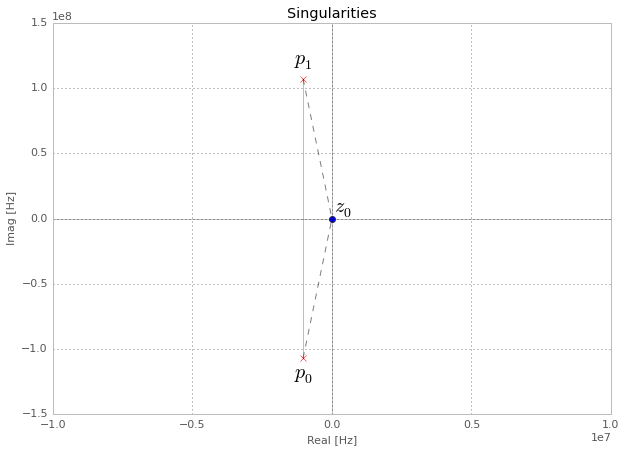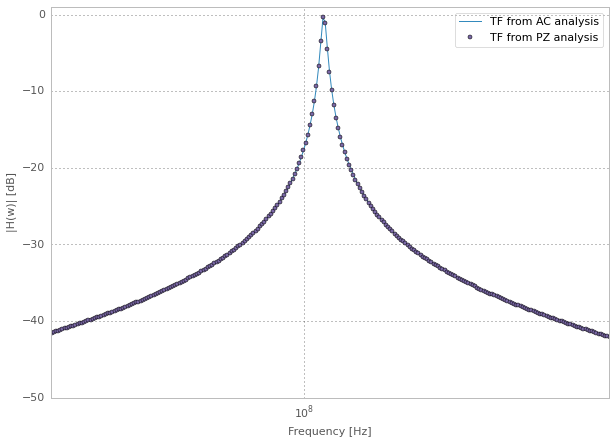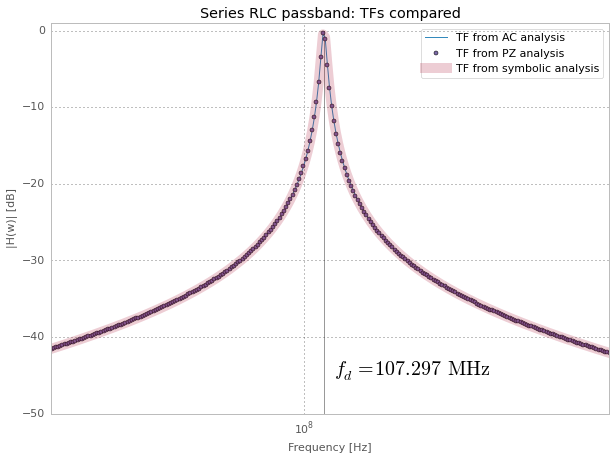# Pole-Zero example¶

Giuseppe Venturini, Thu May 7, 2015

In this short example we will simulate a simple RLC circuit with the ahkab simulator.

In particular, we consider a series resonant RLC circuit. If you need to refresh your knowledge on 2nd filters, you may take a look at this page.

## The plan: what we’ll do¶

### 0. A brief analysis of the circuit¶

This should be done with pen and paper, we’ll just mention the results. The circuit is pretty simple, feel free to skip if you find it boring.

### 1. How to describe the circuit with ahkab¶

We’ll show this:

• from Python,
• and briefly with a netlist deck.

### 2. Pole-zero analysis¶

• We will extract poles and zeros.
• We’ll use them to build input-output transfer function, which we evaluate.

### 3. AC analysis¶

• We will run an AC analysis to evaluate numerically the transfer function.

### 4. Symbolic analysis¶

• We’ll finally run a symbolic analysis as well.
• Once we have the results, we’ll substitute for the real circuit values and verify both AC and PZ analysis.

### 5. Conclusions¶

We will check that the three PZ, AC and Symbolic analysis match!

## The circuit¶

The circuit we simulate is a very simple one:## 0. Theory¶

Once one proves that the current flowing in the only circuit branch in the Laplace domain is given by:

$I(s) = \frac{1}{L}\cdot\frac{s}{s^2 + 2\alpha\cdot s + \omega_0^2}$

Where:

• $$s$$ is the Laplace variable, $$s = \sigma + j \omega$$:
• $$j$$ is the imaginary unit,
• $$\omega$$ is the angular frequency (units rad/s).
• $$\alpha$$ is known as the Neper frequency and it is given by $$R/(2L)$$,
• $$\omega_0$$ is the *(undamped) resonance frequency, equal to $$(\sqrt{LC})^{-1}$$.

It’s easy to show that the pass-band transfer function we consider in our circuit, $$V_{OUT}/V_{IN}$$, has the expression:

$H(s) = \frac{V_{OUT}}{V_{IN}}(s) = k_0 \cdot\frac{s}{s^2 + 2\alpha\cdot s + \omega_0^2}$

Where the coeffiecient $$k_0$$ has value $$k_0 = R/L$$.

Solving for poles and zeros, we get:

• One zero:
• $$z_0$$, located in the origin.
• Two poles, $$p_0$$ and $$p_1$$:
• $$p_{0,1} = - \alpha \pm \sqrt{\alpha^2 - \omega_0^2}$$

## 1. Describe the circuit with ahkab¶

Let’s call ahkab and describe the circuit above.

First we need to import ahkab:

# libraries we need
import ahkab

Populating the interactive namespace from numpy and matplotlib

print "We're using ahkab %s" % ahkab.__version__

We're using ahkab 0.16


Then we create a new circuit object titled ‘RLC bandpass’, which we name bpf from Band-Pass Filter:

bpf = ahkab.Circuit('RLC bandpass')


A circuit is made of, internally, components and nodes. For now, our bpf circuit is empty and really of not much use.

We wish to define our nodes, our components, specifying their connection to the appropriate nodes and inform the circuit instance about the what we did.

It sounds complicated, but it is actually very simple, also thanks to the convenience functions add_*() in the Circuit instances (circuit documentation).

We now add the inductor L1, the capacitor C1, the resistor R1 and the input source V1:

bpf = ahkab.Circuit('RLC bandpass')
# we also give V1 an AC value since we wish to run an AC simulation
# in the following


Notice that:

• the nodes to which they get connected ('in', 'n1', 'out'...) are nothing but strings. If you prefer handles, you can call the create_node() method of the circuit instance bpf (create_node documentation).
• Using the convenience methods add_*, the nodes are not explicitly added to the circuit, but they are in fact automatically taken care of behind the hood.

Now we have successfully defined our circuit object bpf.

Let’s see what’s in there and generate a netlist:

print(bpf)

* RLC bandpass
L1 in n1 1e-06
C1 n1 out 2.2e-12
R1 out 0 13
V1 in 0 type=vdc value=1 vac=1


The above text defines the same circuit in netlist form. It has the advantage that it’s a very concise piece of text and that the syntax resembles (not perfectly yet) that of simulators such as SPICE.

If you prefer to run ahkab from the command line, be sure to check the Netlist syntax doc page and to add the simulation statements, which are missing above.

## 2. PZ analysis¶

The analysis is set up easily by calling ahkab.new_pz(). Its signature is:

ahkab.new_pz(input_source=None, output_port=None, shift=0.0, MNA=None,
outfile=None, x0=u'op', verbose=0)


And you can find the documentation for ahkab.new_pz here.

We will set:

• Input source and output port, to enable the extraction of the zeros.
• the input source is V1,
• the output port is defined between the output node out and ground node (bpf.gnd).
• We need no linearisation, since the circuit is linear. Therefore we set x0 to None.
• I inserted a non-zero shift in the initial calculation frequency below. You may want to fiddle a bit with this value, the algorithm internally tries to kick the working frequency away from the exact location of the zeros, since we expect a zero in the origin, we help the simulation find the zero quickly by shifting away the initial working point.
pza = ahkab.new_pz('V1', ('out', bpf.gnd), x0=None, shift=1e3)
r = ahkab.run(bpf, pza)['pz']


The results are in the pz_solution object r. It has an interface that works like a dictionary.

Eg. you can do:

r.keys()

[u'p0', u'p1', u'z0']


Check out the documentation on pz_solution for more.

Let’s see what we got:

 print('Singularities:')
for x, _ in r:
print "* %s = %+g %+gj Hz" % (x, np.real(r[x]), np.imag(r[x]))

Singularities:
* p0 = -1.03451e+06 -1.07297e+08j Hz
* p1 = -1.03451e+06 +1.07297e+08j Hz
* z0 = -1.44751e-13 +0j Hz


Note that the results are frequencies expressed in Hz (and not angular frequencies in rad/s).

Graphically, we can see better where the singularities are located:

figure(figsize=figsize)
# plot o's for zeros and x's for poles
for x, v in r:
plot(np.real(v), np.imag(v), 'bo'*(x=='z')+'rx'*(x=='p'))
# set axis limits and print some thin axes
xm = 1e6
xlim(-xm*10., xm*10.)
plot(xlim(), [0,0], 'k', alpha=.5, lw=.5)
plot([0,0], ylim(), 'k', alpha=.5, lw=.5)
# plot the distance from the origin of p0 and p1
plot([np.real(r['p0']), 0], [np.imag(r['p0']), 0], 'k--', alpha=.5)
plot([np.real(r['p1']), 0], [np.imag(r['p1']), 0], 'k--', alpha=.5)
# print the distance between p0 and p1
plot([np.real(r['p1']), np.real(r['p0'])],
[np.imag(r['p1']), np.imag(r['p0'])],
'k-', alpha=.5, lw=.5)
# label the singularities
text(np.real(r['p1']), np.imag(r['p1'])*1.1, '$p_1$', ha='center',
fontsize=20)
text(.4e6, .4e7, '$z_0$', ha='center', fontsize=20)
text(np.real(r['p0']), np.imag(r['p0'])*1.2, '$p_0$', ha='center',
va='bottom', fontsize=20)
xlabel('Real [Hz]'); ylabel('Imag [Hz]'); title('Singularities');As expected, we got two complex conjugate poles and a zero in the origin.

The resonance frequency

Let’s check that indeed the (undamped) resonance frequency $$f_0$$ has the expected value from the theory.

It should be:

$f_0 = \frac{1}{2\pi\sqrt{LC}}$

Since we have little damping, $$f_0$$ is very close to the damped resonant frequency in our circuit, given by the absolute value of the imaginary part of either $$p_0$$ or $$p_1$$.

In fact, the damped resonant frequency $$f_d$$ is given by:

$f_d = \frac{1}{2\pi}\sqrt{\alpha^2 -w_0^2}$

Since this is an example and we have Python at our fingertips, we’ll compensate for the frequency pulling due to the damping anyway. That way, the example is analytically correct.

C = 2.2e-12
L = 1e-6
f0 = 1./(2*np.pi*np.sqrt(L*C))
print 'Resonance frequency from analytic calculations: %g Hz' %f0

Resonance frequency from analytic calculations: 1.07302e+08 Hz

alpha = (-r['p0']-r['p1'])/2
a1 = np.real(abs(r['p0'] - r['p1']))/2
f0 = np.sqrt(a1**2 - alpha**2)
f0 = np.real_if_close(f0)
print 'Resonance frequency from PZ analysis: %g Hz' %f0

Resonance frequency from PZ analysis: 1.07292e+08 Hz


That’s alright.

## 3. AC analysis¶

Let’s perform an AC analysis:

aca = ahkab.new_ac(start=1e8, stop=5e9, points=5e2, x0=None)
rac = ahkab.run(bpf, aca)['ac']


Next, we use sympy to assemble the transfer functions from the singularities we got from the PZ analysis.

import sympy
sympy.init_printing()

from sympy.abc import w
from sympy import I
p0, p1, z0 = sympy.symbols('p0, p1, z0')
k = 13/1e-6 # constant term, can be calculated to be R/L
H = 13/1e-6*(I*w + z0*6.28)/(I*w +p0*6.28)/(I*w + p1*6.28)
Hl = sympy.lambdify(w, H.subs({p0:r['p0'], z0:abs(r['z0']), p1:r['p1']}))


We need a function to evaluate the absolute value of a transfer function in decibels.

Here it is:

def dB20(x):
return 20*np.log10(x)


Next we can plot $$|H(\omega)|$$ in dB and inspect the results visually.

figure(figsize=figsize)
semilogx(rac.get_x()/2/np.pi, dB20(abs(rac['vout'])),
label='TF from AC analysis')
semilogx(rac.get_x()/2/np.pi, dB20(abs(Hl(rac.get_x()))), 'o', ms=4,
label='TF from PZ analysis')
legend(); xlabel('Frequency [Hz]'); ylabel('|H(w)| [dB]');
xlim(4e7, 3e8); ylim(-50, 1);## 4. Symbolic analysis¶

Next, we setup and run a symbolic analysis.

We set the input source to be 'V1', in this way, ahkab will calculate all transfer functions, together with low-frequency gain, poles and zeros, with respect to every variable in the circuit.

It is done very similarly to the previous cases:

symba = ahkab.new_symbolic(source='V1')
rs, tfs = ahkab.run(bpf, symba)['symbolic']


Notice how to the 'symbolic' key corresponds a tuple of two objects: the symbolic results and the TF object that was derived from it.

Let’s inspect their contents:

print(rs)

Symbolic simulation results for 'RLC bandpass' (netlist None).
Run on 2015-05-07 04:24:42.
I[L1]    = C1*V1*s/(C1*L1*s**2 + C1*R1*s + 1.0)
I[V1]    = -C1*V1*s/(C1*L1*s**2 + C1*R1*s + 1.0)
VIN  = V1
VN1  = V1*(C1*R1*s + 1.0)/(C1*L1*s**2 + C1*R1*s + 1.0)
VOUT     = C1*R1*V1*s/(C1*L1*s**2 + C1*R1*s + 1.0)

print tfs

Symbolic transfer function results for 'RLC bandpass' (netlist None).
Run on 2015-05-07 04:24:42.
I[L1]/V1:
gain:   C1*s/(C1*L1*s**2 + C1*R1*s + 1.0)
gain0:  0
poles:
0.5*(-C1*R1 + sqrt(C1*(C1*R1**2 - 4.0*L1)))/(C1*L1)
-0.5*(C1*R1 + sqrt(C1*(C1*R1**2 - 4.0*L1)))/(C1*L1)
zeros:
0
I[V1]/V1:
gain:   -C1*s/(C1*L1*s**2 + C1*R1*s + 1.0)
gain0:  0
poles:
0.5*(-C1*R1 + sqrt(C1*(C1*R1**2 - 4.0*L1)))/(C1*L1)
-0.5*(C1*R1 + sqrt(C1*(C1*R1**2 - 4.0*L1)))/(C1*L1)
zeros:
0
VIN/V1:
gain:   1
VN1/V1:
gain:   (C1*R1*s + 1.0)/(C1*L1*s**2 + C1*R1*s + 1.0)
gain0:  1.00000000000000
poles:
0.5*(-C1*R1 + sqrt(C1*(C1*R1**2 - 4.0*L1)))/(C1*L1)
-0.5*(C1*R1 + sqrt(C1*(C1*R1**2 - 4.0*L1)))/(C1*L1)
zeros:
-1/(C1*R1)
VOUT/V1:
gain:   C1*R1*s/(C1*L1*s**2 + C1*R1*s + 1.0)
gain0:  0
poles:
0.5*(-C1*R1 + sqrt(C1*(C1*R1**2 - 4.0*L1)))/(C1*L1)
-0.5*(C1*R1 + sqrt(C1*(C1*R1**2 - 4.0*L1)))/(C1*L1)
zeros:
0


In particular, to our transfer function corresponds:

tfs['VOUT/V1']

{u'gain': C1*R1*s/(C1*L1*s**2 + C1*R1*s + 1.0),
u'gain0': 0,
u'poles': [0.5*(-C1*R1 + sqrt(C1*(C1*R1**2 - 4.0*L1)))/(C1*L1),
-0.5*(C1*R1 + sqrt(C1*(C1*R1**2 - 4.0*L1)))/(C1*L1)],
u'zeros': }


It’s easy to show the above entries are a different formulation that corresponds to the theoretical results we introduced at the beginning of this example.

We’ll do it graphically. First of all, let’s isolate out TF:

Hs = tfs['VOUT/V1']['gain']
Hs

$\frac{C_{1} R_{1} s}{C_{1} L_{1} s^{2} + C_{1} R_{1} s + 1.0}$

We wish to substitute the correct circuit values to R1, L1 and C1 to be able to evaluate numerically the results.

In order to do so, the symbolic_solution class in the results module has a method named as_symbols that takes a string of space-separed symbol names and returns the sympy symbols associated with them (symbolic_solution.as_symbols documentation).

s, C1, R1, L1 = rs.as_symbols('s C1 R1 L1')
HS = sympy.lambdify(w, Hs.subs({s:I*w, C1:2.2e-12, R1:13., L1:1e-6}))


Did we get the same results, let’s sat within a 1dB accuracy?

np.allclose(dB20(abs(HS(rac.get_x()))), dB20(abs(Hl(rac.get_x()))), atol=1)

True


Good.

## 5. Conclusions¶

Let’s take a look at PZ, AC and symbolic results together:

figure(figsize=figsize);  title('Series RLC passband: TFs compared')
semilogx(rac.get_x()/2/np.pi, dB20(abs(rac['vout'])),
label='TF from AC analysis')
semilogx(rac.get_x()/2/np.pi, dB20(abs(Hl(rac.get_x()))), 'o', ms=4,
label='TF from PZ analysis')
semilogx(rac.get_x()/2/np.pi, dB20(abs(HS(rac.get_x()))), '-', lw=10,
alpha=.2, label='TF from symbolic analysis')
vlines(1.07297e+08, *gca().get_ylim(), alpha=.4)
text(7e8/2/np.pi, -45, '$f_d = 107.297\\, \\mathrm{MHz}$', fontsize=20)
legend(); xlabel('Frequency [Hz]'); ylabel('|H(w)| [dB]');
xlim(4e7, 3e8); ylim(-50, 1);I hope this example helped show how to use ahkab and in particular how to perform PZ, AC and symbolic analysis. If it also cleared up some doubts, great!

Please remember this is an experimental simulator and you may find bug... it’s getting better but we’re not really ready for prime time yet: please report any and all bugs you may encounter on the issue tracker.

This document was written with Jupiter running with a Python kernel (project formerly named IPython). You can find it here: Jupyter/IPython and you may access the whole notebook, which will allow you to download and modify this example.

Have fun!